## A 600-kg car traveling at 30.0 m/s is going around a curve having a radius of 120 m that is banked at an angle of 25.0°. The coefficient of

Question

A 600-kg car traveling at 30.0 m/s is going around a curve having a radius of 120 m that is banked at an angle of 25.0°. The coefficient of static friction between the car’s tires and the road is 0.300. What is the magnitude of the force exerted by friction on the car?

in progress 0
6 months 2021-08-04T19:41:19+00:00 1 Answers 74 views 0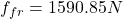Explanation:

Here the total force at the horizontal components will be equal to the centripetal force on the car. So we will have: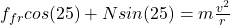(1)

• f(fr) is the friction force
• N is the normal force

Now, the sum of forces at the vertical direction is equal to 0.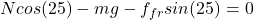(2)

Let’s combine (1) and (2) to find f(fr)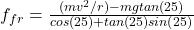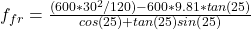I hope it helps you!